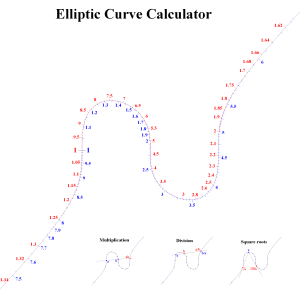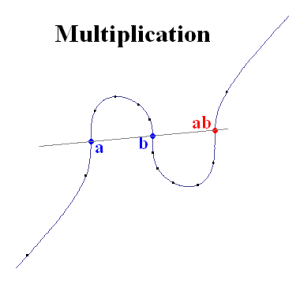# Elliptic curve calculator

What’s the cheapest calculator you know of? Basic calculators are much cheaper than their scientific counterparts, and you can probably get one for £2. A slide rule is even cheaper: you could strap together two plastic rulers with logarithmic scales for about 20p — a saving of an order of magnitude. Pretty impressive for a couple of sliding scales.

We can do much better than this, though. You can print a fully functional calculator, capable of multiplication, division and extraction of square roots, on a single sheet of A4 (or A3 for ‘double precision’!) paper. This is probably an order of magnitude again cheaper than a regular slide rule, costing about 2 pence.A sheet of paper capable of multiplication, division and square root computation, with similar precision to a slide rule but for one tenth of the price.

Clicking the above image will link to a full-size version, which you can print off and use. Note that it has two scales, red and blue, running in opposite directions on an elliptic (non-singular cubic) curve. The scales are reciprocals of each other; for any point labelled x in red and y in blue, xy = 1.

To multiply two numbers together, find them (ignoring decimal places; 10x and x are represented by the same point) in the same colour and draw a straight line through them. This will intersect the elliptic curve in a third point. Read the result off on the oppositely-coloured scale; this is the product of the two numbers!Find two numbers (a and b) in the same colour (blue), and project a line through them to meet the curve in a third point. Read this off in the opposite colour (red) to obtain the product, ab.

Similarly, you can divide and compute square-roots, as shown in the pictorial instructions at the foot of the image. It’s very simple to use, but to explain why it works is much more difficult; it relies on some advanced algebraic geometry and group theory typically encountered in degree-level mathematics. If you really want to know why, continue reading. But be warned: the mathematics gets quite complicated.

It all boils down to the fact that the points on an elliptic curve form an Abelian group. This has a similar structure to the real numbers, which means that we can interchange between the two. (This is not so straightforward, requiring a transcendental function related to the Weierstrass P-function. I used repeated interval bisection and polynomial interpolation to obtain a good approximation instead.)

We define an operation on the points of the elliptic curve. We’ll represent this with a funky symbol such as @. For three collinear points on the curve, A, B and C, we say that A @ B @ C = e, where e is the identity element. It’s pretty obvious that B @ A @ C = e, as well, since the order of points on the line is unimportant. In other words, A @ B = B @ A — the group operation is commutative. It suffices only to show that A @ (B @ C) = (A @ B) @ C — associativity — before we can treat @ like addition or multiplication, which are themselves equivalent due to the exponential function. Proving associativity is possible with a lovely theorem called Cayley-Bacharach.

In my elliptic curve calculator, I have used @ to emulate multiplication. Division is rather routine, since it’s just reverse-engineering multiplication. Finding the point B with a tangent intersecting the curve at A is equivalent to solving A @ B @ B = e, so B is the square root of the reciprocal of A.

The geometry of elliptic curves has applications in advanced cryptography, where it offers superior security compared with ordinary RSA (Rivest-Shamir-Adleman). It’s very easy with repeated squaring to quickly compute the nth power (B = A @ A @ … @ A) of a point A on the curve. However, given A and B, it is very difficult to reverse-engineer the process to obtain n. This is known as the discrete logarithm problem. With some effort, it can be adapted into a cryptosystem similar to RSA, but in the setting of elliptic curves instead of modular arithmetic.

Elliptic curves were also the tools used by Andrew Wiles to prove (most of) the Tanayama-Shimura Conjecture and thus Fermat’s Last Theorem. The simplest proof of Poncelet’s porism also involves elliptic curves.

This entry was posted in Uncategorized. Bookmark the permalink.

### 16 Responses to Elliptic curve calculator

1.David Stay says:

When I tried out your calculator I found that for division you get 10a/b. For example 1/5=2

•apgoucher says:

Well spotted. For a real number x, the numbers {…, 0.1x, x, 10x, 100x, …} are all represented by the same point on the elliptic curve. It’s a consequence of the Weierstrass P-function being doubly periodic — everything wraps around.

2.apgoucher says:

Richard Guy (one of the early developers of combinatorial game theory) informs me that he had done something essentially equivalent about 60 years ago, entitled ‘a single scale nomogram’.

•Anonymous says:

“2315. A Single-Scale Nomogram,” Richard K. Guy, The Mathematical Gazette, Vol. 37, No. 319 (Feb., 1953), p. 39, MAA. Article Stable URL: http://www.jstor.org/stable/3609499

3.Jason says:

This is pretty cool! To find square roots, you look at tangent lines to other points; I don’t think the diagram explains this very well.

•apgoucher says:

Indeed. You start with your straightedge on a point x, and rotate it about x until it is tangent to the elliptic curve at a second point. This is either sqrt(x) or sqrt(10x). On a similar topic, the points of inflection of the curve correspond to 1, cbrt(10) and cbrt(100).

4.Isaac Kuo says:

I don’t understand the math of this elliptic curve, but now I want to make a spherical ball version, using a gnomonic projection. Imagine an orb Coke bottle, with this curve projected on it. Also, a great circle is marked.

You fill the orb up to the great circle. Now, you can calculate by tilting the orb.

5.Andrew Carlotti says:

6.Andy says:

That’s a really cool calculator. I’d like to understand ist construction. How did you create the curve, e.g. which equation did you use, and how did you calculate the marks?

•Andy says:

Well, the equation is y³=x³-x, or some scaled and/or translated copy thereof. While tickmarking a cubic curve (y=x³-x) is fairly simple, I don’t get how it is done with elliptic curves. E.g. why is the origin set to the left zero point (root?) instead of the center? Why are the other zero points somehow depend on sqrt(2) (log(100*sqrt(2) = 2,1505 at the center)?

•apgoucher says:

(Note that the points on this curve represent equivalence classes of positive reals, where x is equivalent to y if and only if x/y is 10^k for some integer k.)

Any of the three inflection points can be used as the multiplicative identity, 1. The reason for not choosing the middle one is so that the ‘blind spots’ for the red and blue scales (the ranges of numbers that are beyond the edge of the displayed portion of the curve) are not the same, and ideally disjoint.

The other two inflection points end up being 10 ^ (1/3) and 10 ^ (2/3).

Now, if you start with the ‘1’ inflection point (which, because we’ve identified powers of 10, also represents 10, 100, 1000, and so forth) and apply the square-root method — drawing a line through ‘1’ which is tangent to the curve at a distinct point — we get the point which we can label sqrt(10). Applying the square-root method to this point gives us two points, 10^(1/4) and 10^(3/4). Repeatedly applying this produces every dyadic rational power of 10. These are a dense subset of the reals, i.e. we can get arbitrarily close to any given [equivalence class of] reals by repeatedly taking square-roots.

If you don’t like this iterative approach, then you can do this analytically. You’ll find this easiest if your elliptic curve is in Weierstrass form, in which case you can use the P-function:

https://en.wikipedia.org/wiki/Weierstrass%27s_elliptic_functions

7.Steven Tague says:

The missing red and blue values would be needed for some problem. Even if the operations could exchange red and blue, the answer to some problems are still going to be off the chart, unless you have the whole curve labeled.. Then you have to find an infinite true straightedge, and a place to lay the chart on plane surface. My Log-Log-Deci-Trig slide rule has not gaps and can do cube roots too.